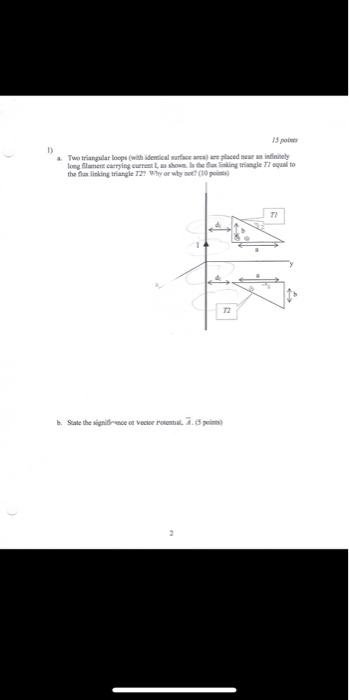Question(a). N, they are not equal. The flux passing through T1 is greater than T2. This is because Magnetic field of an infinite wire is inversely proportional to the distance and T1 has a larger area near the wire.

(b). With vector potential, we can evaluate magnetic field, the current and electric fieldHowever, it has not physical significance. It is merely a computational tool.

#### Earn Coins

Coins can be redeemed for fabulous gifts.

Similar Homework Help Questions
• ### 15 points Two triangular loops (with idenical suface areallaced near an infininely long Elament c...15 points Two triangular loops (with idenical suface areallaced near an infininely long Elament carrying eTR L as showm Is the ining trangle TI equal to the lax linking triangle 122 y or wim co p TI 72 15 points Two triangular loops (with idenical suface areallaced near an infininely long Elament carrying eTR L as showm Is the ining trangle TI equal to the lax linking triangle 122 y or wim co p TI 72